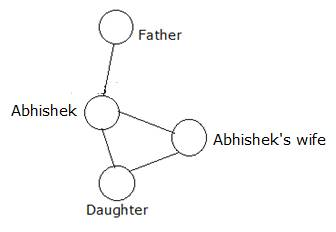## Blood Relation – Practice set 12

1. Looking at a portrait of a man, Harsh said, “His mother is the wife of my father’s son. Brothers and sisters I have none.” At whose portrait was Harsh looking?

a) son

b) Brother

c) cousin

d) Uncle

a) son

A

Explanation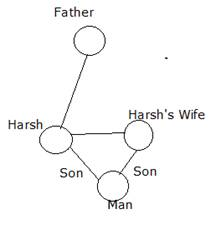P + Q means P is sister of Q

P # Q means P is husband of Q

P \$ Q means P is the daughter of Q

P % Q means P is the mother of Q

P @ Q means P is the brother of Q

2. Which among the given expressions indicate that J is the wife of F?

a) P \$ J % R + K % F

b) F @ R + K + P % J

c) J % P + K @ R \$ F

d) J % P # K + R \$ F

e) R @ P + K \$ F @ J

c) J % P + K @ R \$ F

C

Explanation

(c)

+ Sister

# Husband

\$ Daughter

÷ Mother

@ Brother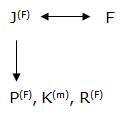3. What should come in place of the question mark, to establish that B is the child of K in the expression?

T % K # F % L + N ? B

a) Only %

b) Either + or @

c) Only #

d) Either @ or %

e) Only +

b) Either + or @

B

Explanation

(b)

+ Sister

# Husband

\$ Daughter

÷ Mother

@ Brother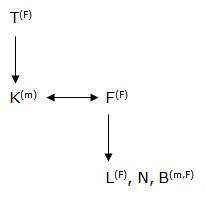D.4-5) Read the following information carefully and answer the question which follows:

‘A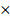B’ means ‘A is the father of B’.

‘A + B’ means ‘A is the daughter of B’.

‘A ÷ B’ means ‘A is the son of B’.

‘A – B’ means ‘A is the sister of B’.

4. Which of the following expressions is definitely true if the given expressions ‘R < P’ as well as ‘S > Q’ are definitely true?

a) P > Q = R ≤ T < S

b) S > T ≥ R > Q < P

c) Q > R ≤ T > P ≥ S

d) S > T ≥ R > Q > P

e) None of these

a) P > Q = R ≤ T < S

A

Explanation

(a)

Both R < P and S > Q True

R < P => P > Q = R => P > R

S > Q => Q = R ≤ T < S => Q < S

5. What will come in place of question mark to establish that P is the son-in-law of S in the following expression?

PQ + R – T ? S

a) +

b)c)

d) ÷

e) Either + or ÷

e) Either + or ÷

E

Explanation

(e)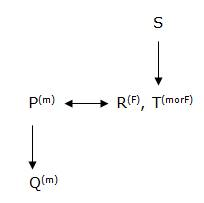× Father

+ Daughter

÷ Son

­ Sister

Either

‘+’ or ‘÷’

D.6-8) Study the following information carefully to answer the given questions:

B is sister of A. A is father of G. H is the only son of F. F is only son-in-law of A. G is the mother of H.

6. How is A related to H?

a) Uncle

b) Father

c) Paternal grandfather

d) Maternal grandfather

e) None of these

d) Maternal grandfather

D

Explanation

(d)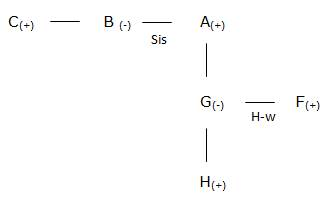Maternal grandfather

7. How is G related to B?

a) Brother

b) Niece

c) Sister

d) Nephew

e) None of these

b) Niece

B

Explanation

(b)Niece

8. If C is the husband of B, then how is A related to C?

a) Father

b) Brother-in-law

c) Mother

d) Brother

e) None of these

b) Brother-in-law

B

Explanation

(b)Brother-in-law

9. Pointing to a photograph, Gopi said, “She is the daughter of my grandfather’s only son.” How is Gopi related to the girl in the photograph?

a) Father

b) Brother

c) Cousin

d) Uncle

b) Brother

B

Explanation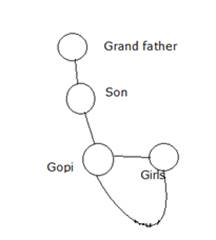10) Pointing towards a girl, Abhishek says, “This girl is the daughter of only child of my father.” What is the relation of Abhishek’s wife to that girl?

a) Mother

b) Sister

c) Aunt

d) Sister-in-law